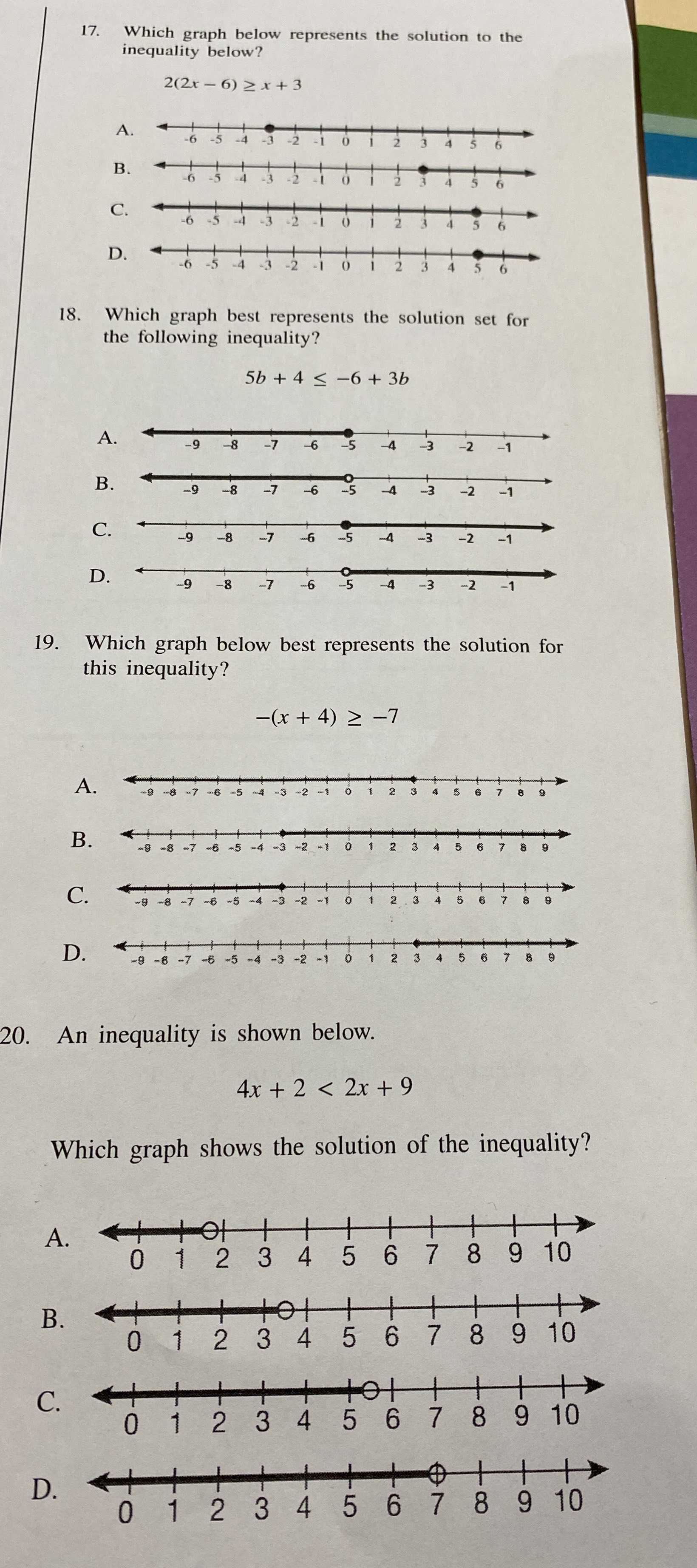### ¿Todavía tienes preguntas de matemáticas?

Pregunte a nuestros tutores expertos
Algebra
Pregunta17. Which graph below represents the solution to the inequality below?

$$2 ( 2 x - 6 ) \geq x + 3$$

18. Which graph best represents the solution set for the following inequality?

$$5 b + 4 \leq - 6 + 3 b$$

19. Which graph below best represents the solution for this inequality?

$$- ( x + 4 ) \geq - 7$$

20. An inequality is shown below.

$$4 x + 2 < 2 x + 9$$

Which graph shows the solution of the inequality?